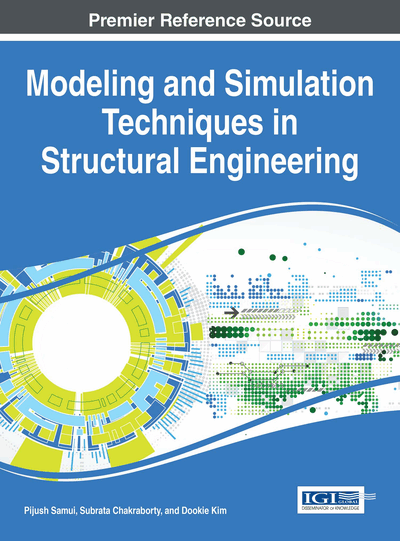# Seismic Reliability Analysis in the Framework of Metamodelling Based Monte Carlo Simulation

Shyamal Ghosh (Indian Institute of Engineering Science and Technology (IIEST), Shibpur, India), Soham Mitra (Indian Institute of Engineering Science and Technology (IIEST), Shibpur, India), Swarup Ghosh (Indian Institute of Engineering Science and Technology (IIEST), Shibpur, India) and Subrata Chakraborty (Indian Institute of Engineering Science and Technology (IIEST), Shibpur, India)
DOI: 10.4018/978-1-5225-0588-4.ch006

## Abstract

A comparative study of various metamodelling approaches namely the least squares method (LSM), moving least squares method (MLSM) and artificial neural network (ANN) based response surface method (RSM) are presented to demonstrate the effectiveness to approximate the nonlinear dynamic response of structure required for efficient seismic reliability analysis (SRA) of structures. The seismic response approximation by the LSM, MLSM and ANN based RSMs are explained with a brief note on the important issue of ground motion bin generation. The procedure adopted herein for SRA is based on the dual response surface approach. In doing so, the repetition of seismic intensity for SRA at different intensity levels is avoided by including this as one of the predictors in the seismic response prediction model. A nonlinear SDOF system has been taken up to elucidate the effectiveness of various metamodels in SRA.
Chapter Preview
Top

## Introduction

Seismic Reliability Analysis (SRA) of structures is basically a structural reliability analysis problem in which the limit state of interest is the difference between seismic demand (D) and capacity (C) considering uncertainty due to earthquake motions, structural properties, physical damage, economic and human losses etc. The problem can be envisaged as a time dependent structural reliability analysis problem in which the limit state of interest can be expressed as,

(1) where,andare the variables governing the capacity and demand and t is the time parameter. The computation of probability that the limit state function is negative means to evaluate the seismic risk of the structure i.e.

(2)

Thus, the seismic risk analysis is the evolution of the following multi-dimensional integral to obtain the probability of failure (Pf),

(3) where X is an ‘n’ dimensional vector having variables involvingand, fZ(X) is the joint probability density function (pdf) of the involved random variables. The exact computation of the above is often computationally demanding. In fact, the joint pdf ofis hardly available in closed form. Various approximations are usually adopted to obtain the probability of exceeding a response parameter for different limit states of damage.

## Complete Chapter List

Search this Book:
Reset